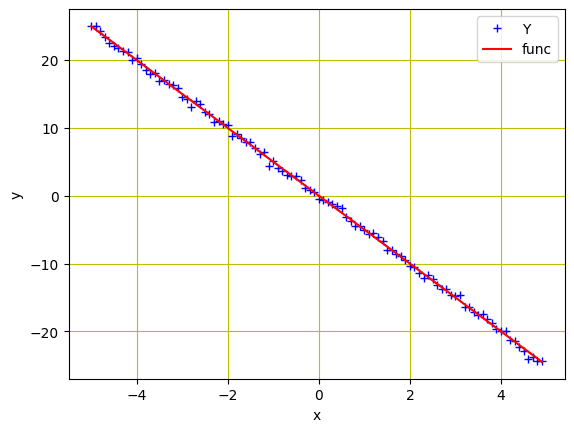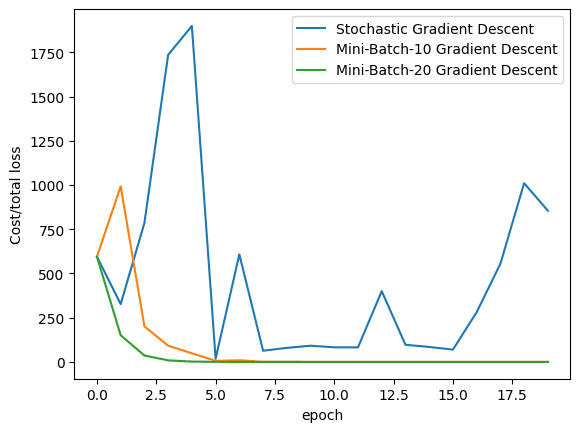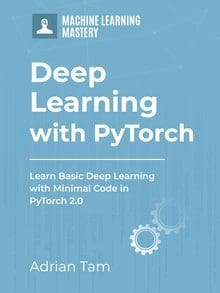SALE! Use code blackfriday for 40% off everything!
Hurry, sale ends soon! Click to see the full catalog.

Mini-batch gradient descent is a variant of gradient descent algorithm that is commonly used to train deep learning models. The idea behind this algorithm is to divide the training data into batches, which are then processed sequentially. In each iteration, we update the weights of all the training samples belonging to a particular batch together. This process is repeated with different batches until the whole training data has been processed. Compared to batch gradient descent, the main benefit of this approach is that it can reduce computation time and memory usage significantly as compared to processing all training samples in one shot.

DataLoader is a module in PyTorch that loads and preprocesses data for deep learning models. It can be used to load the data from a file, or to generate synthetic data.

In this tutorial, we will introduce you to the concept of mini-batch gradient descent. You will also get to know how to implement it with PyTorch DataLoader. Particularly, we’ll cover:

• Implementation of Mini-Batch Gradient Descent in PyTorch.
• The concept of DataLoader in PyTorch and how we can load the data with it.

Kick-start your project with my book Deep Learning with PyTorch. It provides self-study tutorials with working code.

Let’s get started.Picture by Yannis Papanastasopoulos. Some rights reserved.

## Overview

This tutorial is in six parts; they are

• Preparing Data and the Linear Regression Model
• Build Dataset and DataLoader Class
• Plotting Graphs for Comparison

It all starts with loading the data when you plan to build a deep learning pipeline to train a model. The more complex the data, the more difficult it becomes to load it into the pipeline. PyTorch DataLoader is a handy tool offering numerous options not only to load the data easily, but also helps to apply data augmentation strategies, and iterate over samples in larger datasets. You can import DataLoader class from torch.utils.data, as follows.

There are several parameters in the DataLoader class, we’ll only discuss about dataset and batch_size. The dataset is the first parameter you’ll find in the DataLoader class and it loads your data into the pipeline. The second parameter is the batch_size which indicates the number of training examples processed in one iteration.

## Preparing Data and the Linear Regression Model

Let’s reuse the same linear regression data as we produced in the previous tutorial:

Same as in the previous tutorial, we initialized a variable X with values ranging from $-5$ to $5$, and created a linear function with a slope of $-5$. Then, Gaussian noise is added to create the variable Y.

We can plot the data using matplotlib to visualize the pattern:Data points for regression model

Next, we’ll build a forward function based on a simple linear regression equation. We’ll train the model for two parameters ($w$ and $b$). So, let’s define a function for the forward pass of the model as well as a loss criterion function (MSE loss). The parameter variables w and b will be defined outside of the function:

### Want to Get Started With Deep Learning with PyTorch?

Take my free email crash course now (with sample code).

Click to sign-up and also get a free PDF Ebook version of the course.

## Build Dataset and DataLoader Class

Let’s build our Dataset and DataLoader classes. The Dataset class allows us to build custom datasets and apply various transforms on them. The DataLoader class, on the other hand, is used to load the datasets into the pipeline for model training. They are created as follows.

## Training with Stochastic Gradient Descent and DataLoader

When the batch size is set to one, the training algorithm is referred to as stochastic gradient descent. Likewise, when the batch size is greater than one but less than the size of the entire training data, the training algorithm is known as mini-batch gradient descent. For simplicity, let’s train with stochastic gradient descent and DataLoader.

As before, we’ll randomly initialize the trainable parameters $w$ and $b$, define other parameters such as learning rate or step size, create an empty list to store the loss, and set the number of epochs of training.

In SGD, we just need to pick one sample from the dataset in each iteration of training. Hence a simple for loop with a forward and backward pass is all we needed:

Putting everything together, the following is a complete code to train the model, namely, w and b:

## Training with Mini-Batch Gradient Descent and DataLoader

Moving one step further, we’ll train our model with mini-batch gradient descent and DataLoader. We’ll set various batch sizes for training, i.e., batch sizes of 10 and 20. Training with batch size of 10 is as follows:

And, here is how we’ll implement the same with batch size of 20:

Putting all together, the following is the complete code:

## Plotting Graphs for Comparison

Finally, let’s visualize how the loss decreases in all the three algorithms (i.e., stochastic gradient descent, mini-batch gradient descent with batch size of 10, and with batch size of 20) during training.As we can see from the plot, mini-batch gradient descent can converge faster because we can make more precise update to the parameters by calculating the average loss in each step.

Putting all together, the following is the complete code:

## Summary

In this tutorial, you learned about mini-batch gradient descent, DataLoader, and their implementation in PyTorch. Particularly, you learned:

• Implementation of mini-batch gradient descent in PyTorch.
• The concept of DataLoader in PyTorch and how we can load the data with it.
• How to implement stochastic gradient descent with PyTorch DataLoader.
• How to implement mini-batch gradient descent with PyTorch DataLoader.

## Get Started on Deep Learning with PyTorch!#### Learn how to build deep learning models

...using the newly released PyTorch 2.0 library

Discover how in my new Ebook:
Deep Learning with PyTorch

It provides self-study tutorials with hundreds of working code to turn you from a novice to expert. It equips you with
tensor operation, training, evaluation, hyperparameter optimization, and much more...